# RD Sharma Solutions for Class 12 Maths Chapter 14 Differentials, Errors and Approximations

## RD Sharma Solutions for Class 12 Maths Chapter 14 – Free PDF Download

RD Sharma Solutions for Class 12 Chapter 14 – Differentials, Errors and Approximations is provided here for students to study and prepare for their exams easily. RD Sharma books have been prescribed for years as a complete source of information to CBSE students, to develop their analytical skills. For which the RD Sharma Solutions for Class 12 Chapter 14 have proven to be essential for learning the syllabus and developing the confidence that is required to face their exams. The RD Sharma Solutions explain the steps with precision, without missing out on essential aspects of solving a question.

Further, the RD Sharma Solutions are curated by subject experts at BYJU’S according to the latest CBSE Syllabus and guidelines. Informative tips and tricks are provided for students to fetch the maximum marks in their exams.

The 14th Chapter Differentials, Errors and Approximations of RD Sharma Solutions for Class 12 Maths explains the geometrical meanings of differentials. The main aim of these RD Sharma Class 12 Solutions formulated by BYJU’S experts is to provide a fundamental aspect of Maths, which in turn, helps the students to understand every concept clearly.

Some of the essential topics of this chapter are listed below.

• Definition of differentials
• Meaning of differentials
• Absolute error
• Relative error
• Percentage error
• Geometrical meaning of differentials
• Steps or algorithm of finding the geometrical meaning of differentials
• Finding the approximate value using differentials
• Some of the important results on differentials

## RD Sharma Solutions Class 12 Maths Chapter 14 Differentials, Errors and Approximations: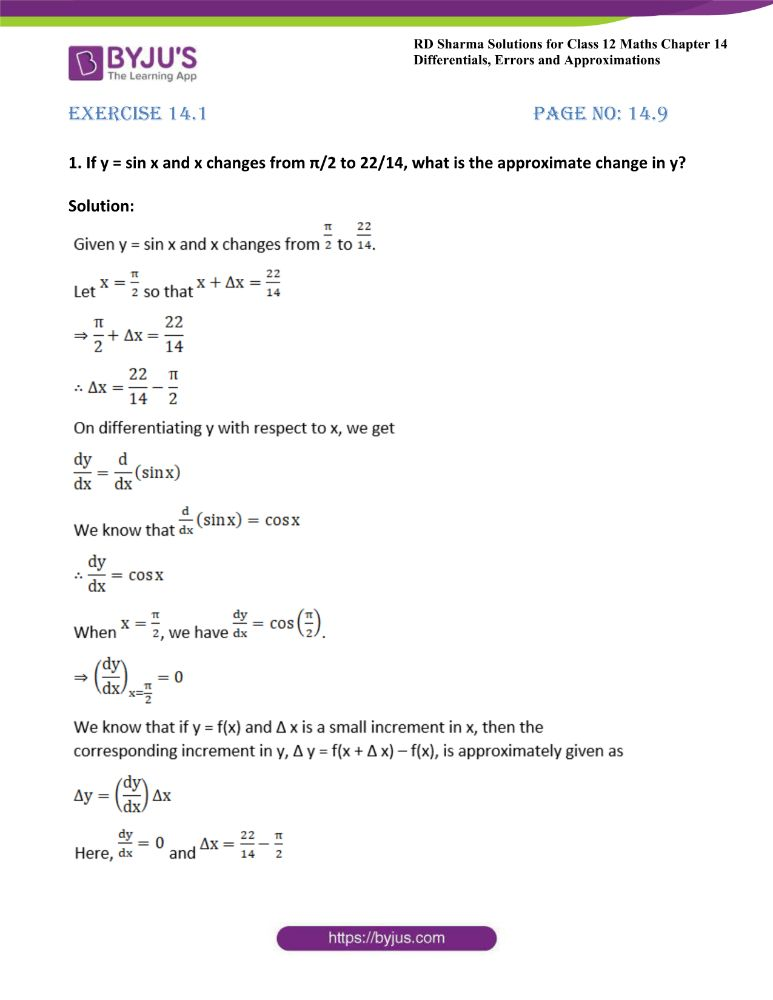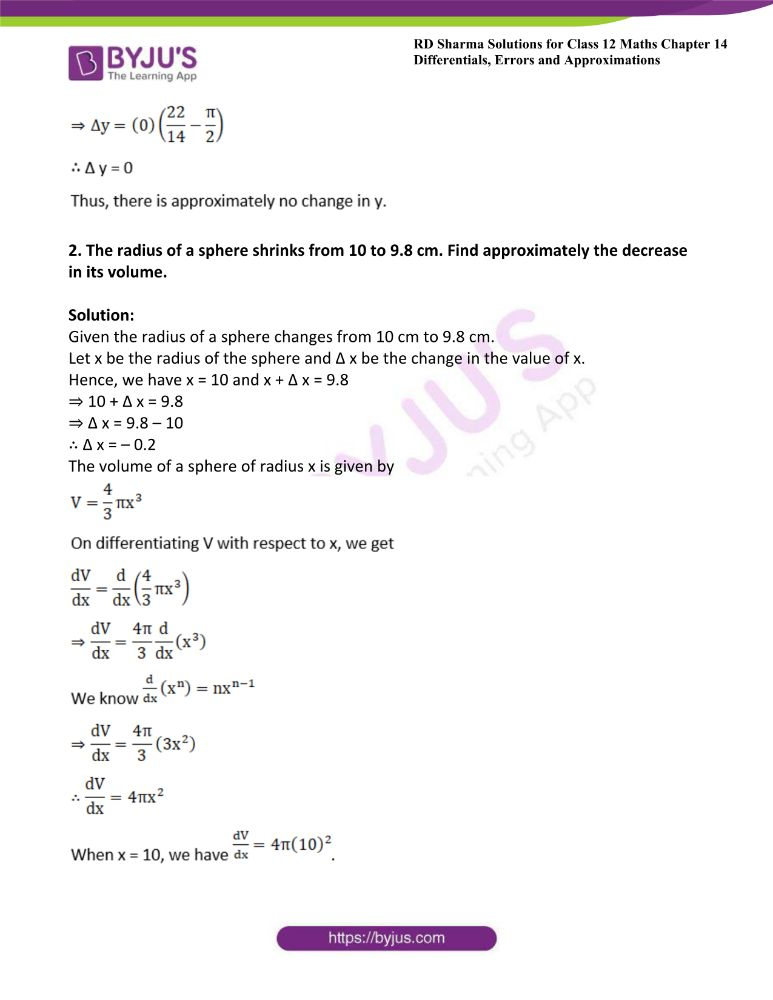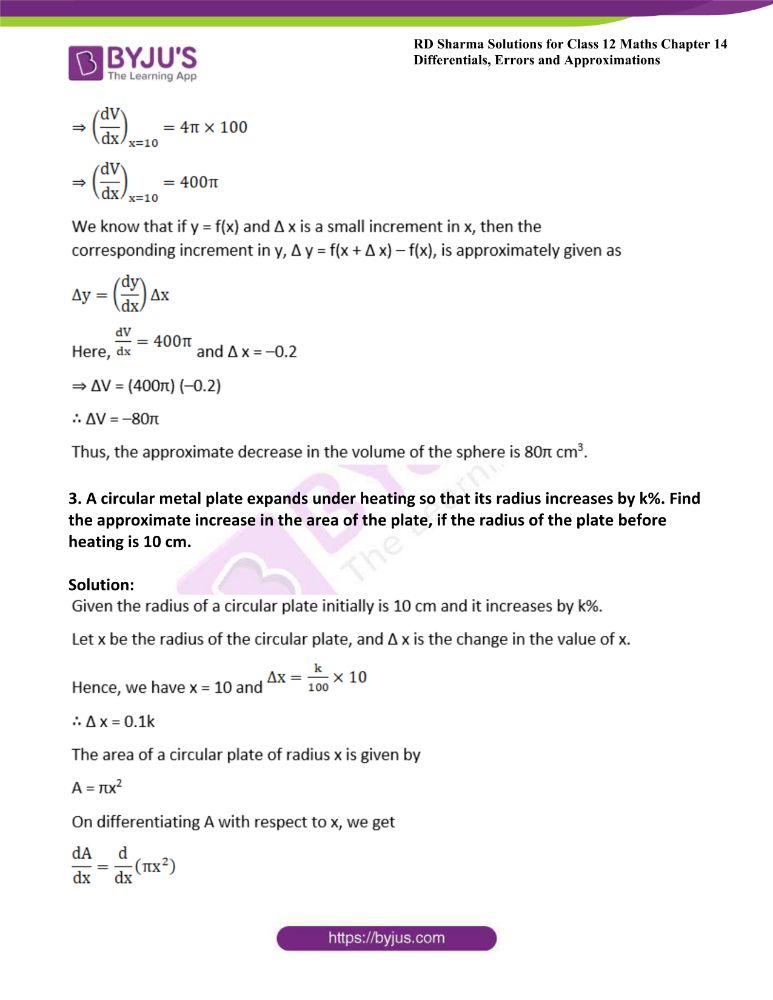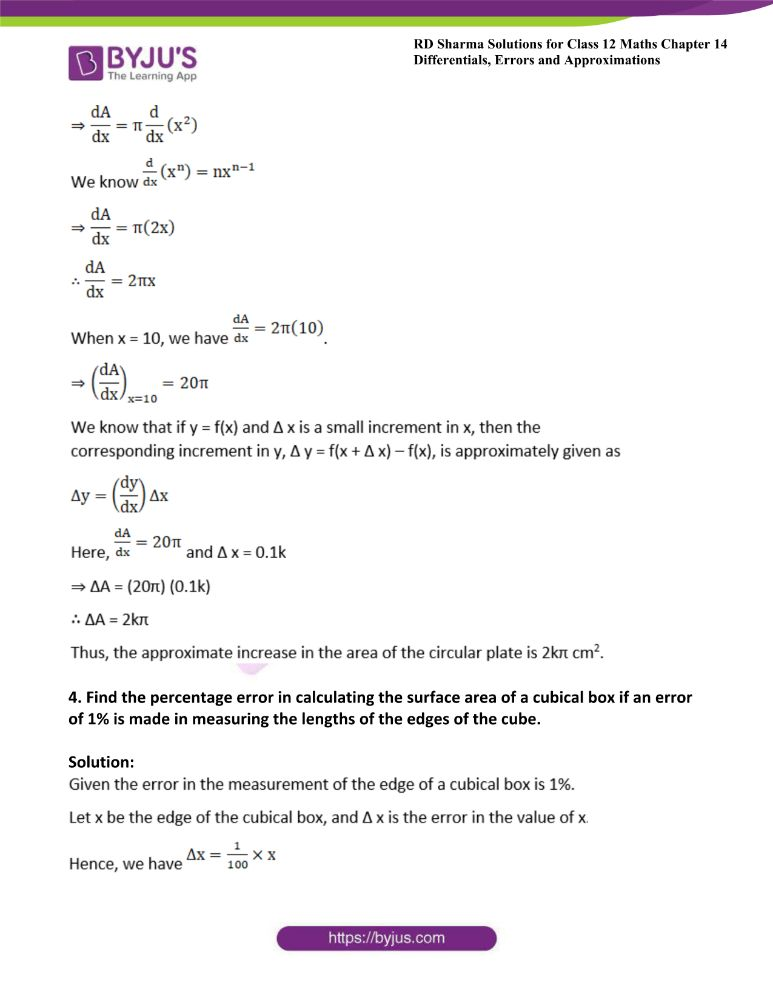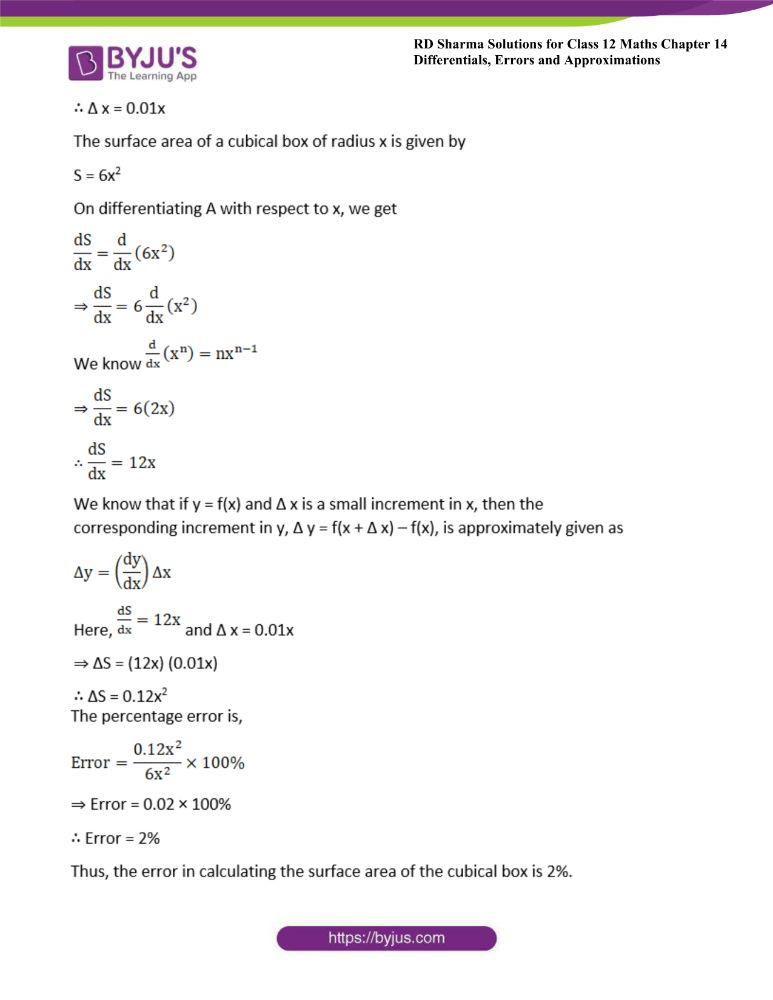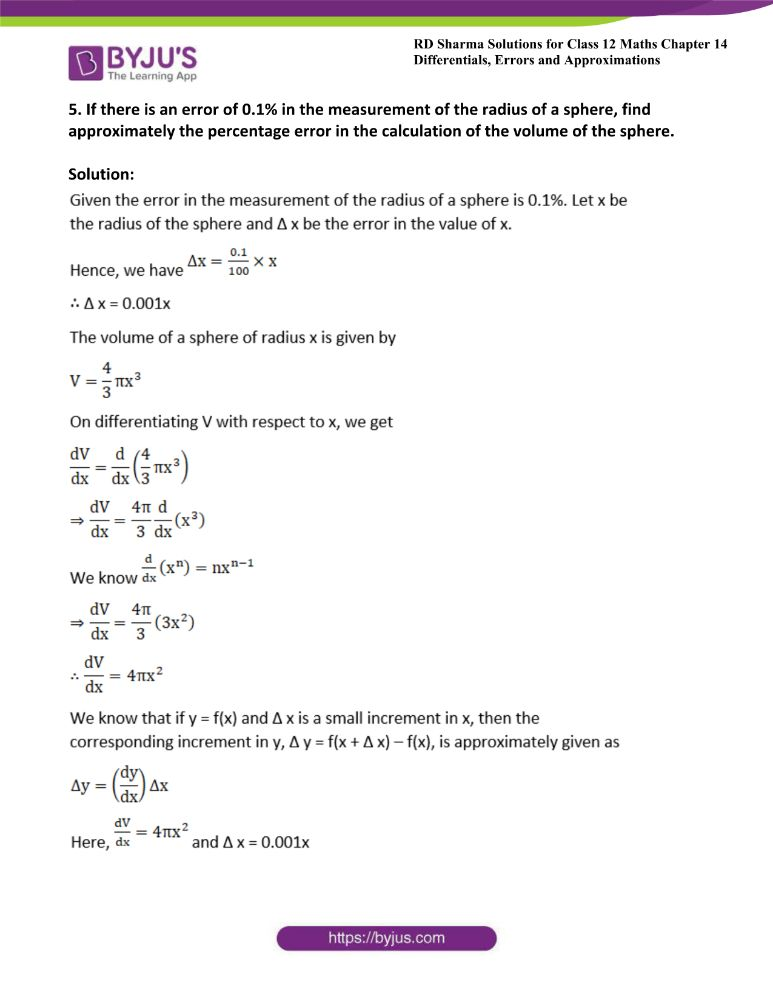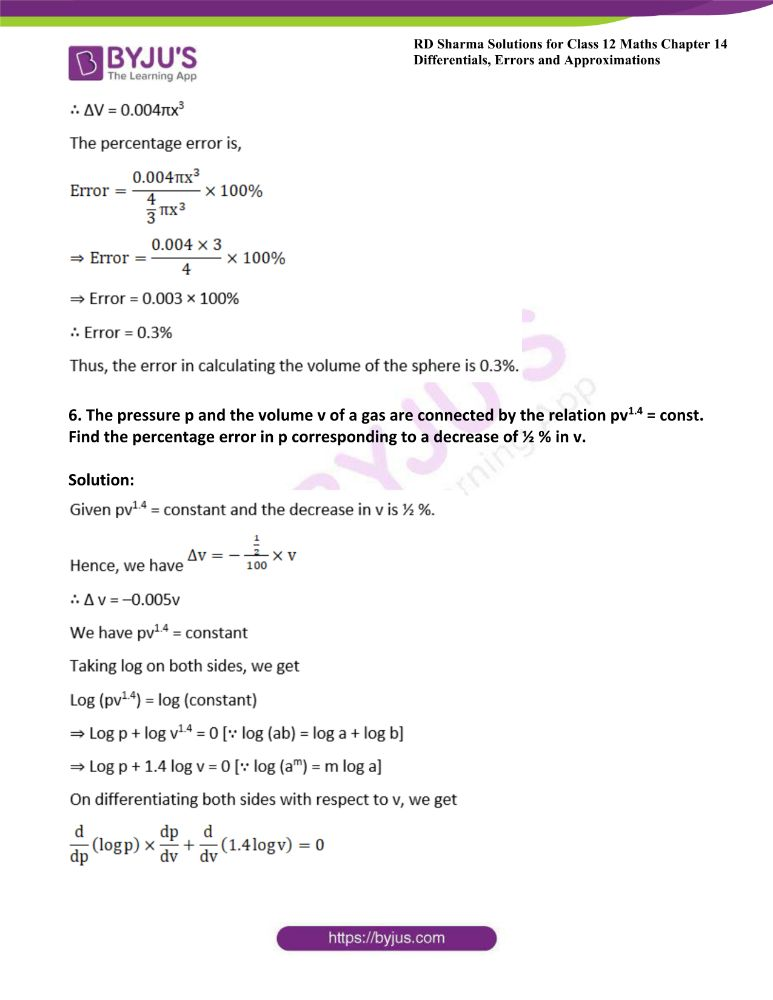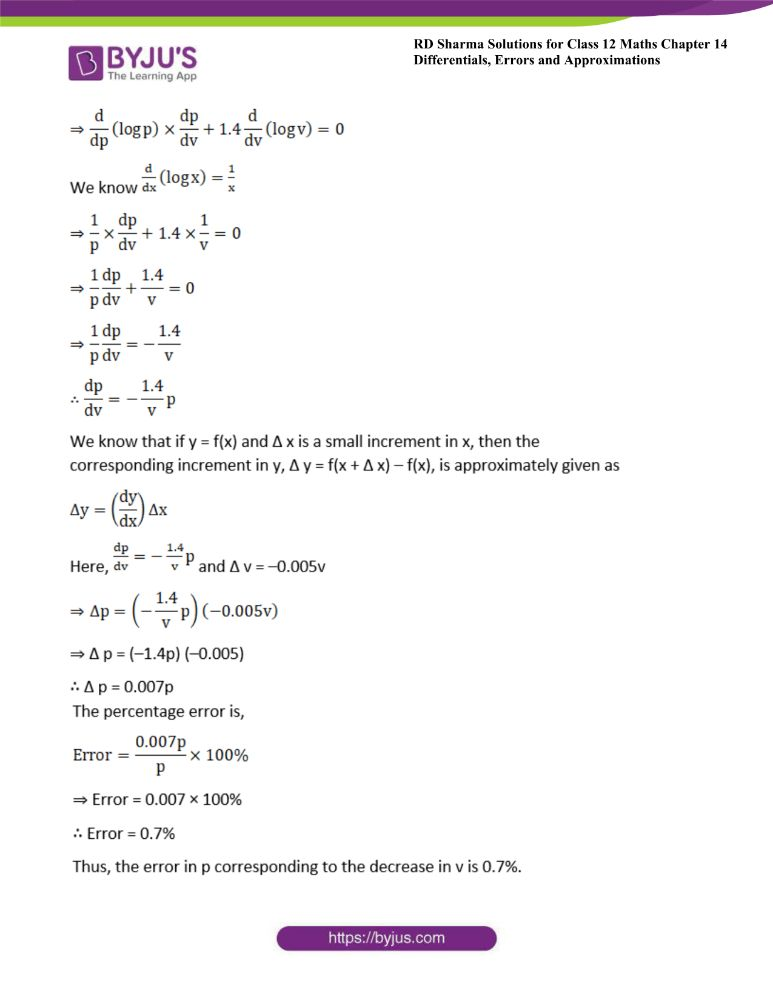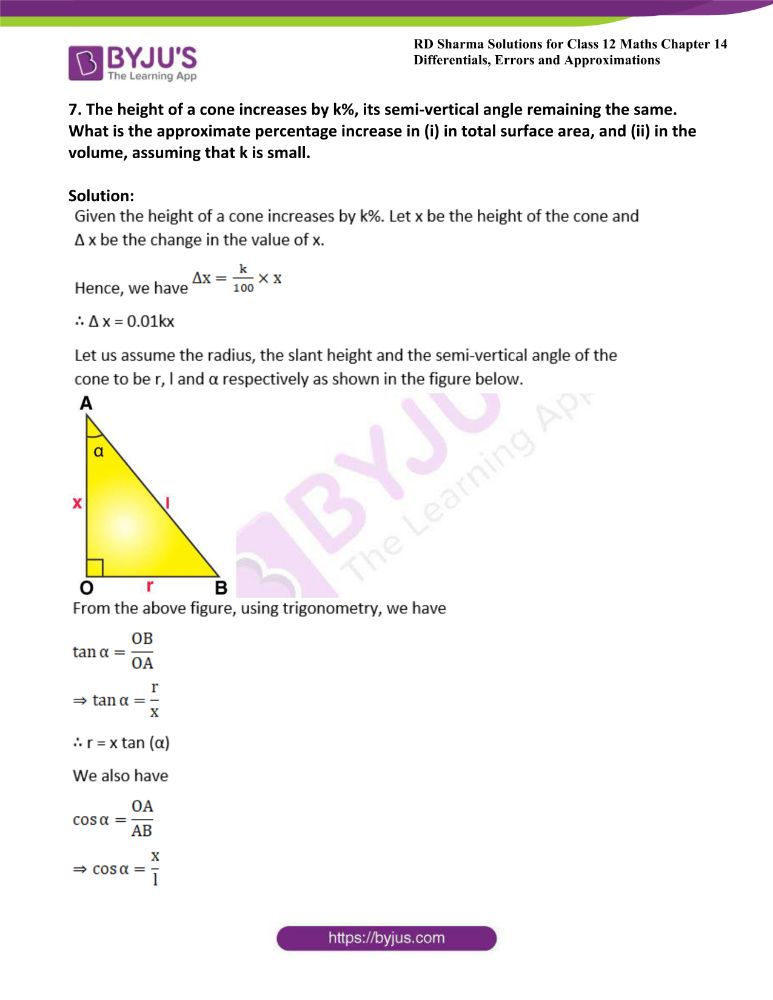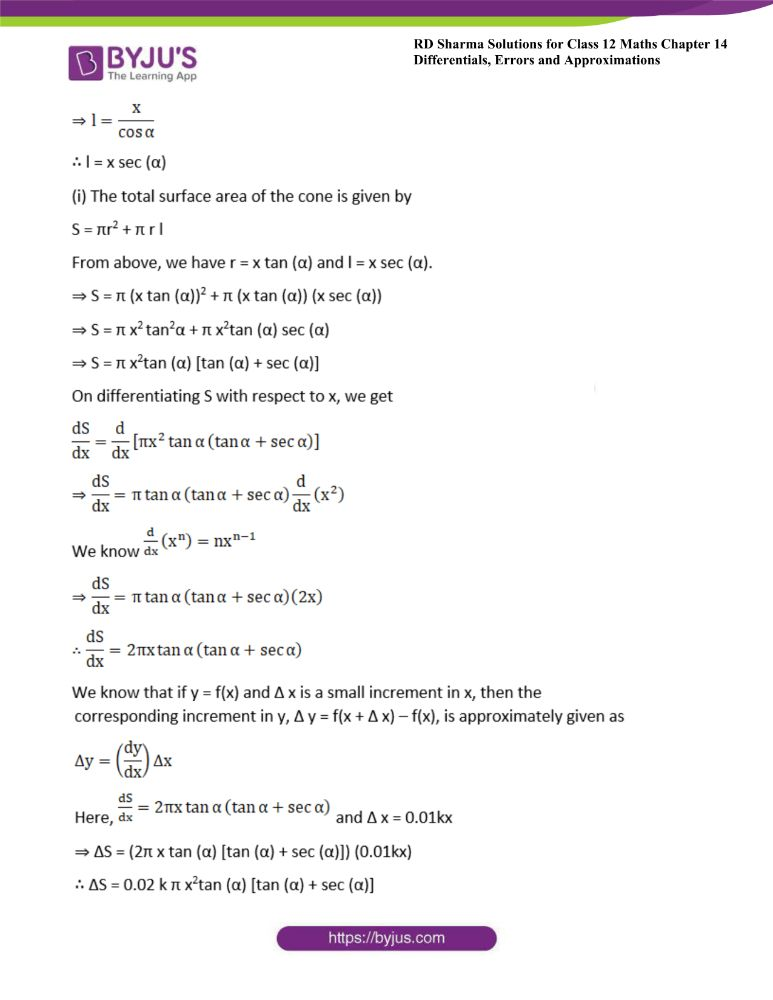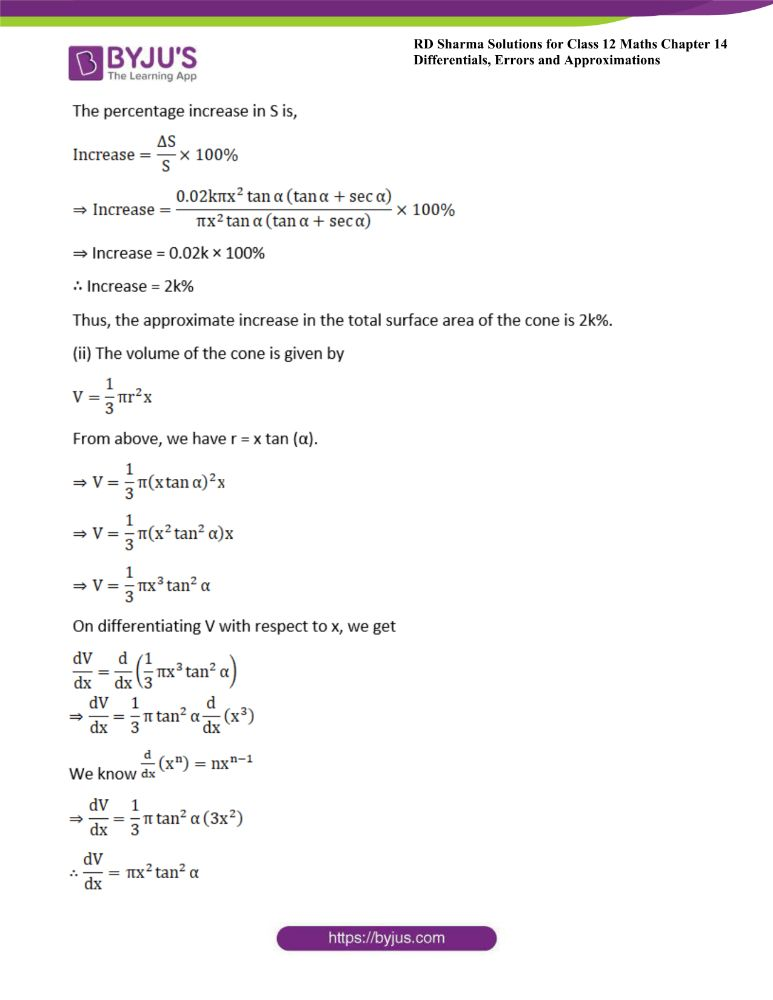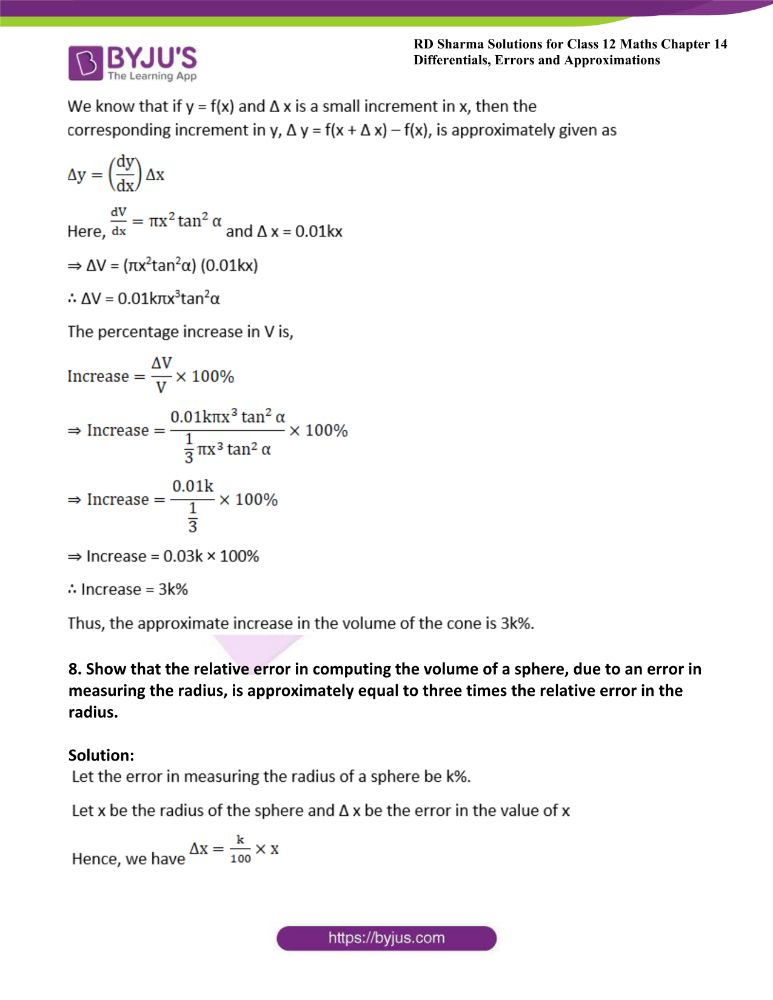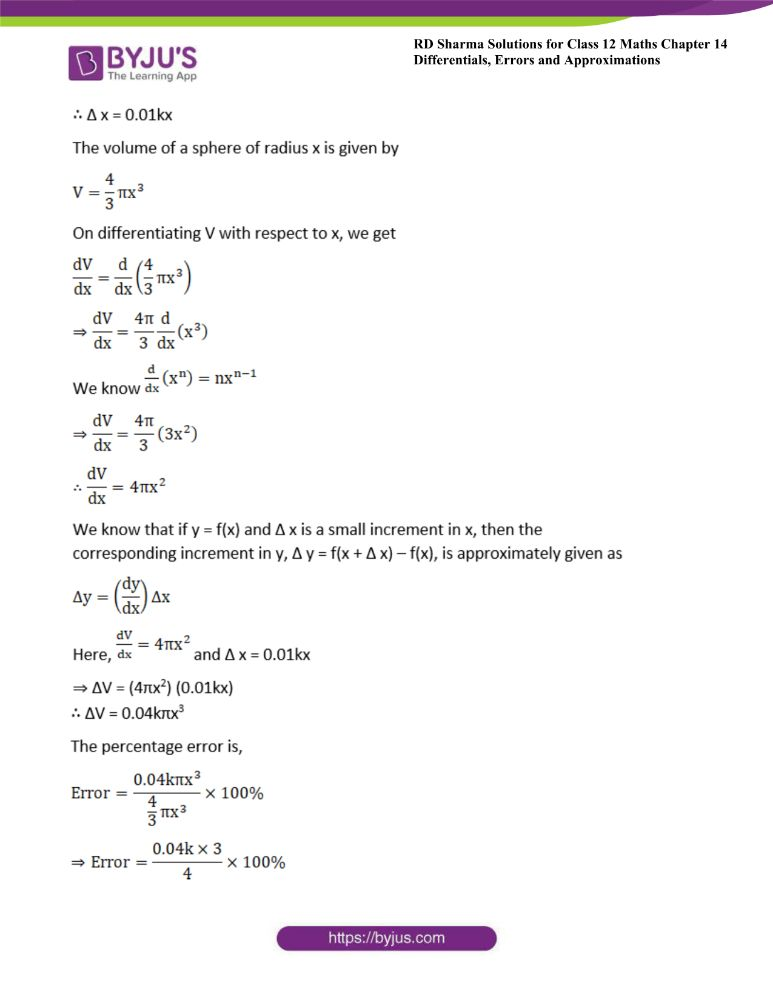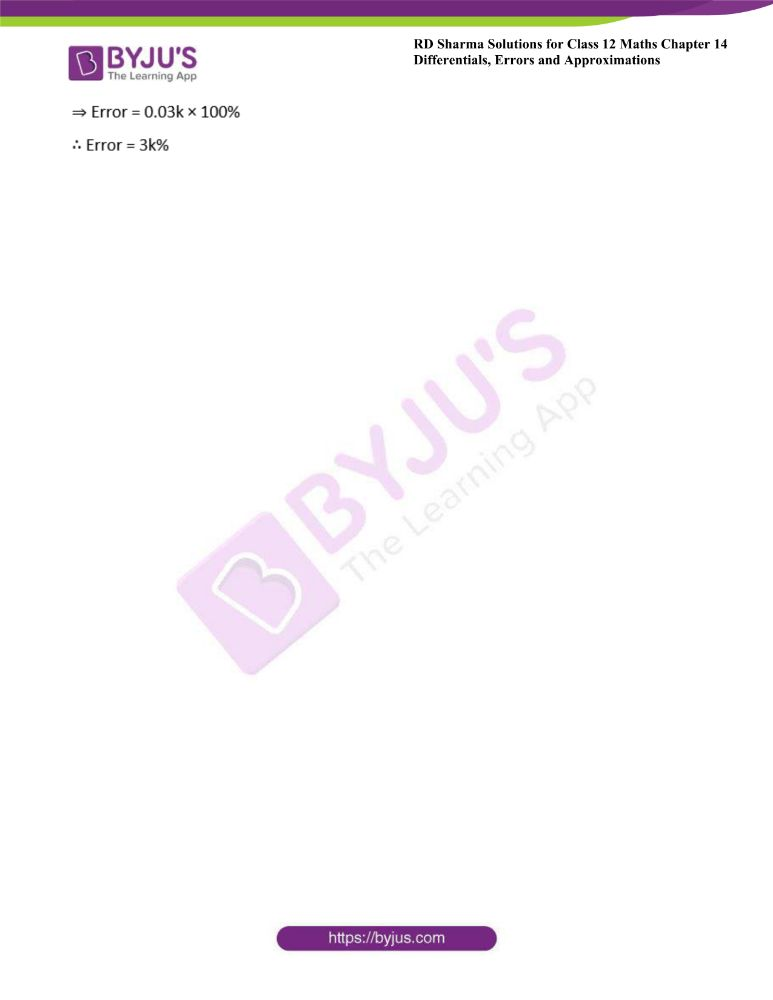### Access answers to Maths RD Sharma Solutions For Class 12 Chapter 14 – Differentials, Errors and Approximations

Exercise 14.1 Page No: 14.9

1. If y = sin x and x changes from π/2 to 22/14, what is the approximate change in y?

Solution: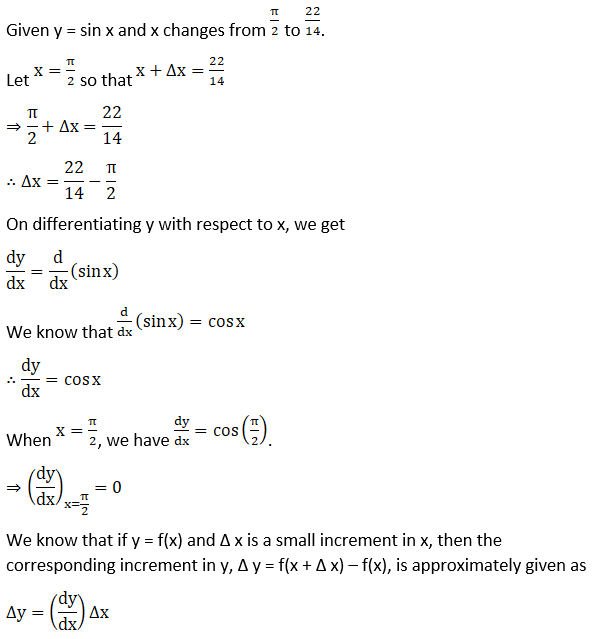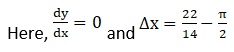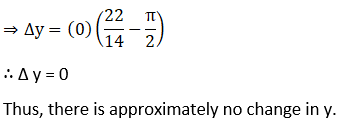2. The radius of a sphere shrinks from 10 to 9.8 cm. Find approximately the decrease in its volume.

Solution:

Given the radius of a sphere changes from 10 cm to 9.8 cm.

Let x be the radius of the sphere and Δ x be the change in the value of x.

Hence, we have x = 10 and x + Δ x = 9.8

⇒ 10 + Δ x = 9.8

⇒ Δ x = 9.8 – 10

∴ Δ x = – 0.2

The volume of a sphere of radius x is given by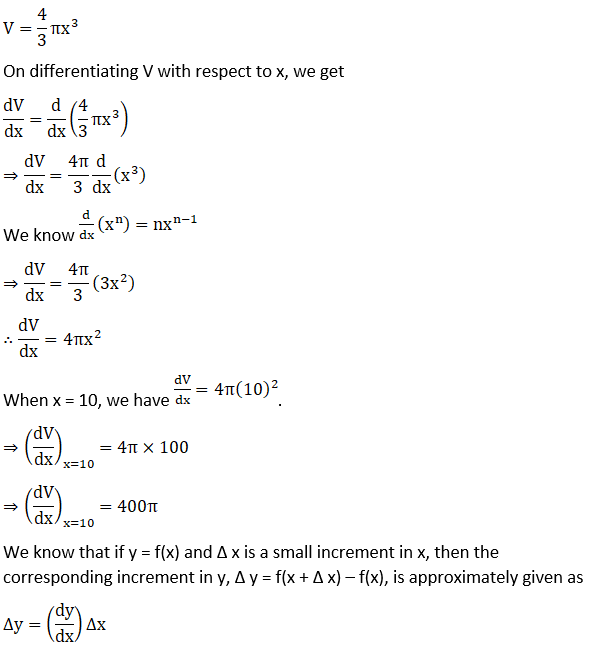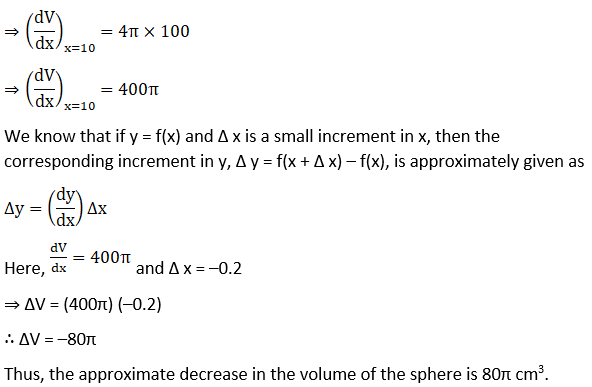3. A circular metal plate expands under heating so that its radius increases by k%. Find the approximate increase in the area of the plate, if the radius of the plate before heating is 10 cm.

Solution: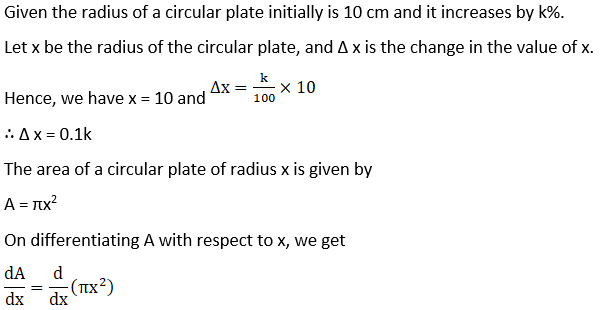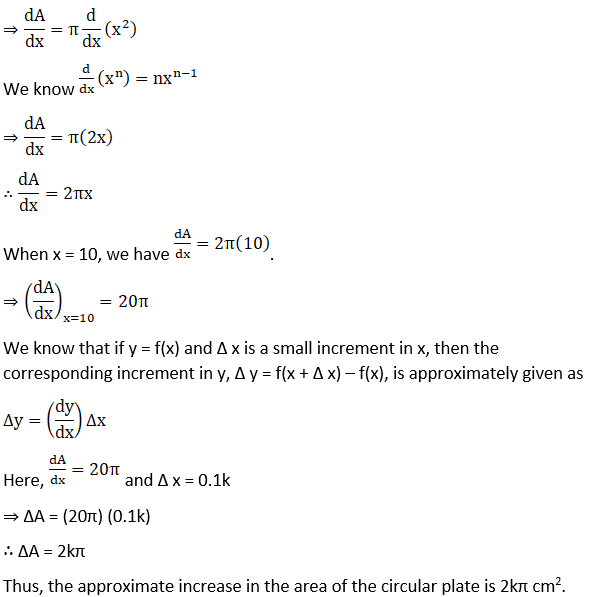4. Find the percentage error in calculating the surface area of a cubical box if an error of 1% is made in measuring the lengths of the edges of the cube.

Solution: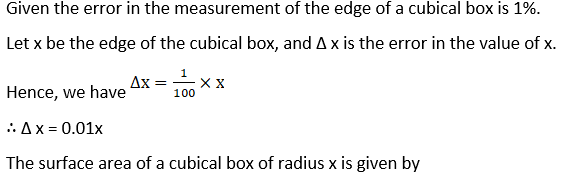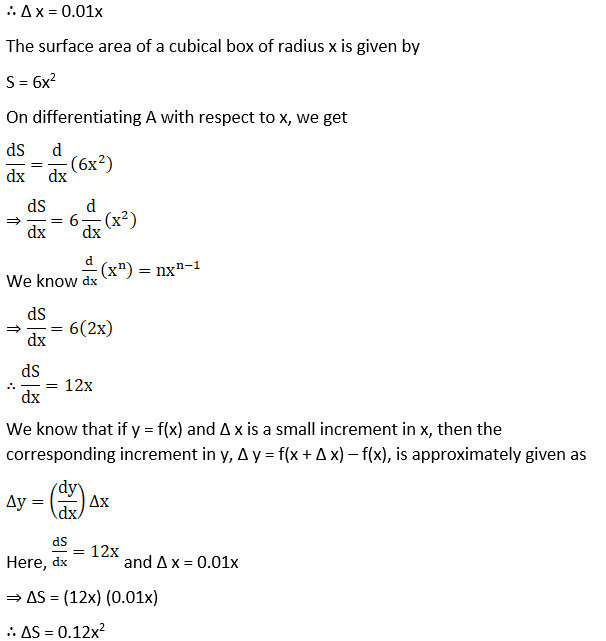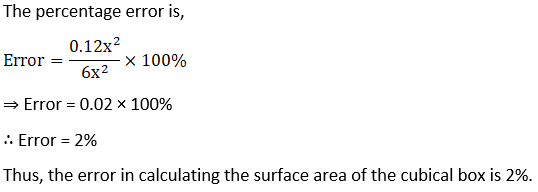5. If there is an error of 0.1% in the measurement of the radius of a sphere, find approximately the percentage error in the calculation of the volume of the sphere.

Solution: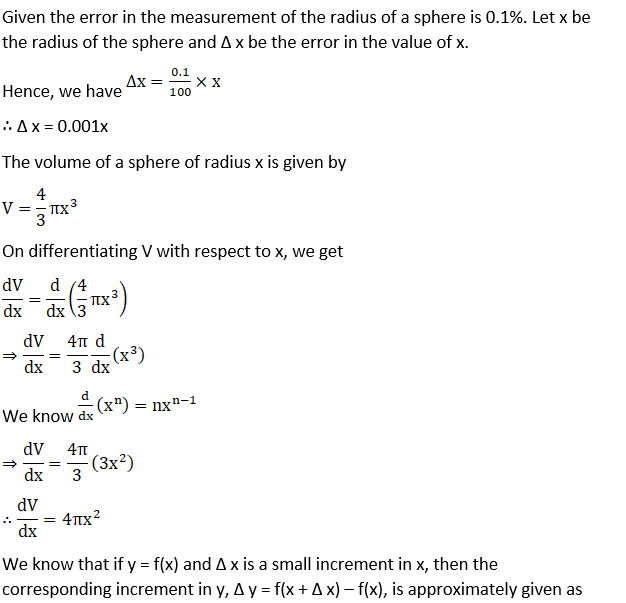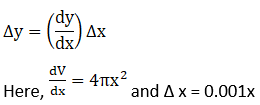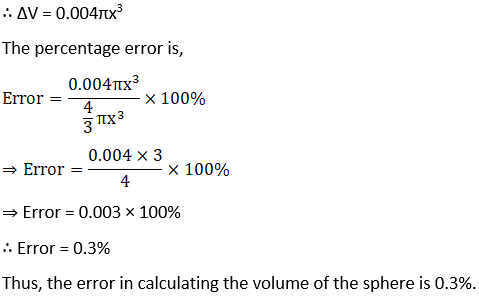6. The pressure p and the volume v of a gas are connected by the relation pv1.4 = const. Find the percentage error in p corresponding to a decrease of ½ % in v.

Solution: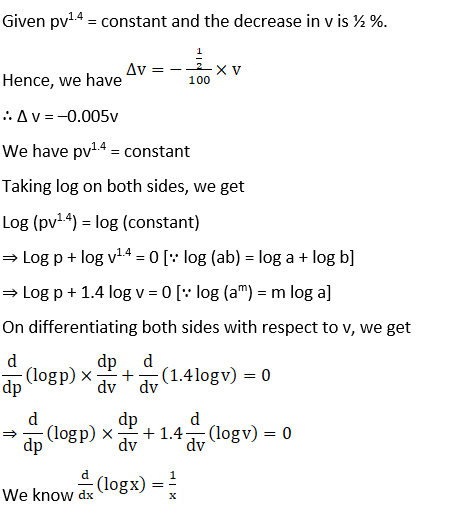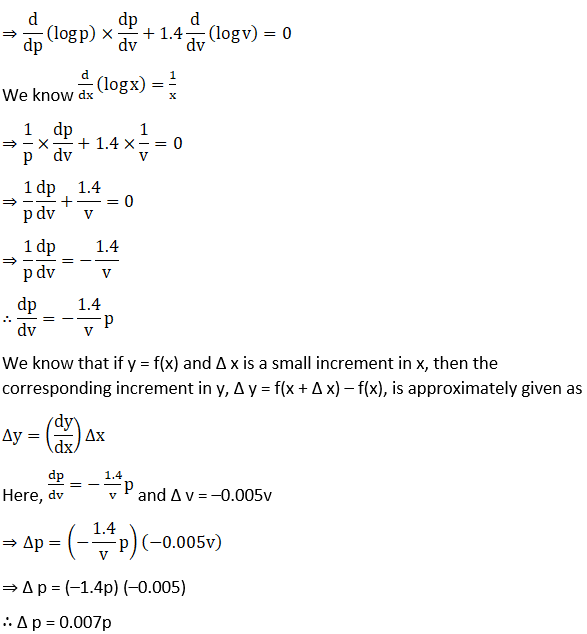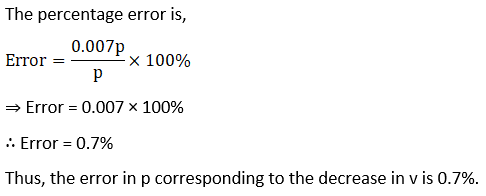7. The height of a cone increases by k%, its semi-vertical angle remaining the same. What is the approximate percentage increase in (i) in total surface area, and (ii) in the volume, assuming that k is small.

Solution: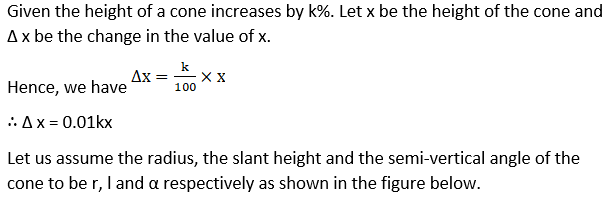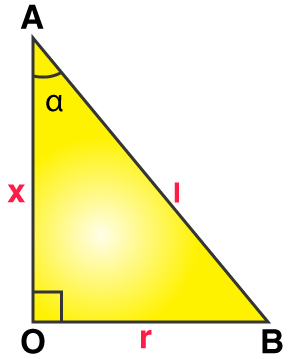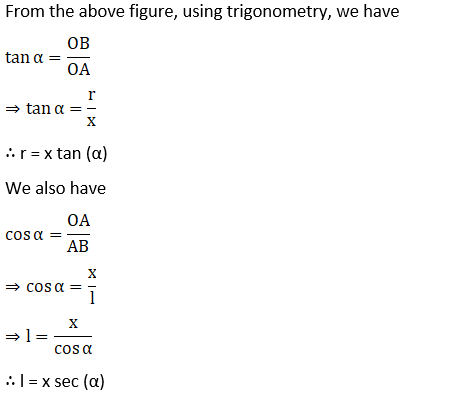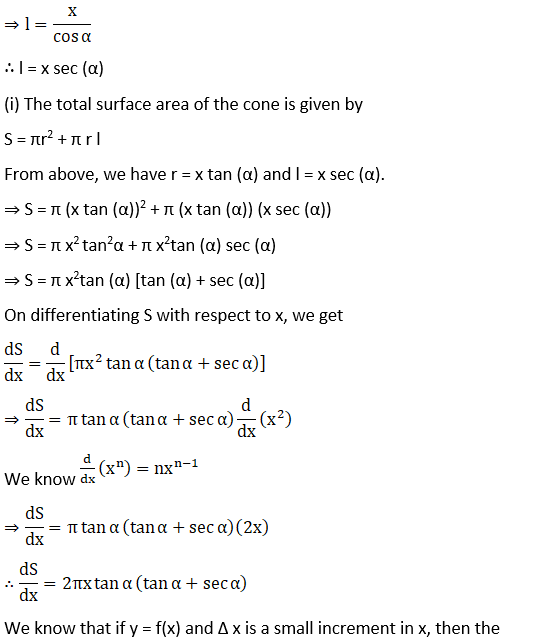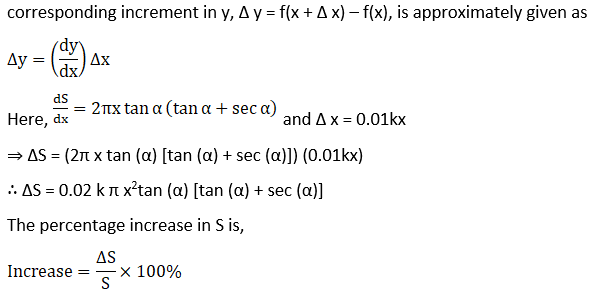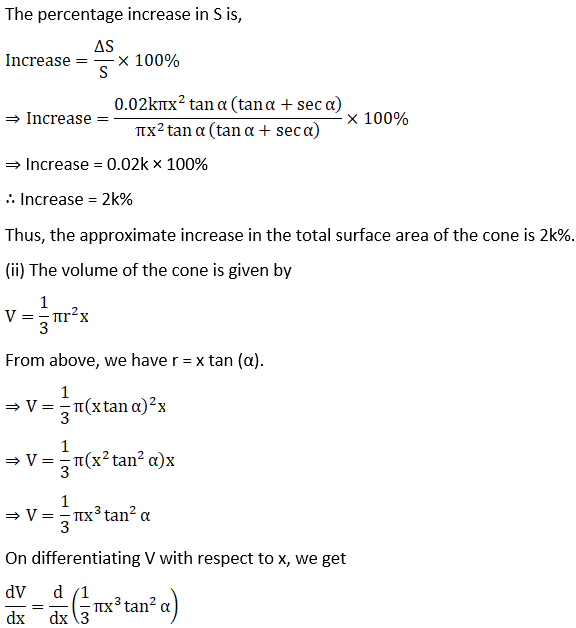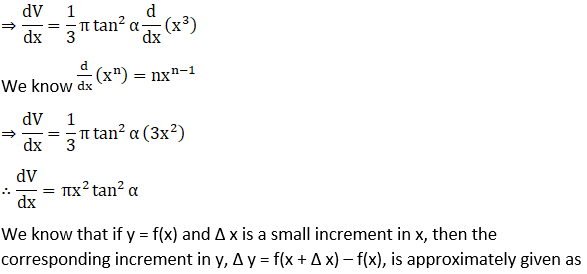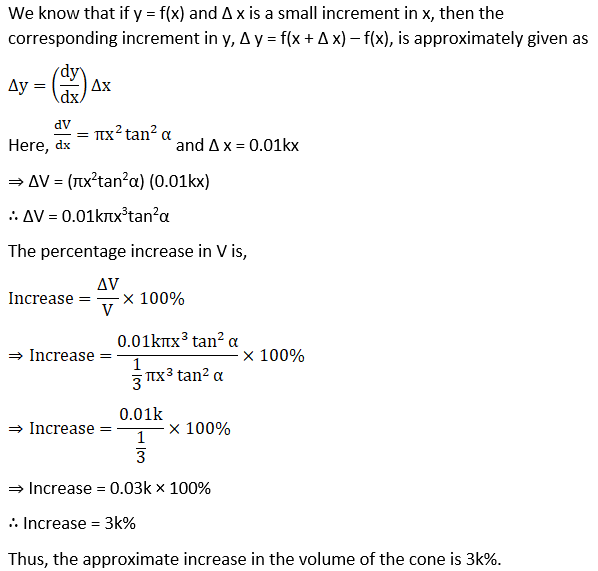8. Show that the relative error in computing the volume of a sphere, due to an error in measuring the radius, is approximately equal to three times the relative error in the radius.

Solution: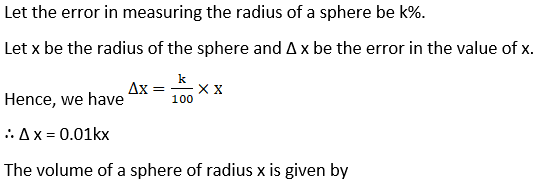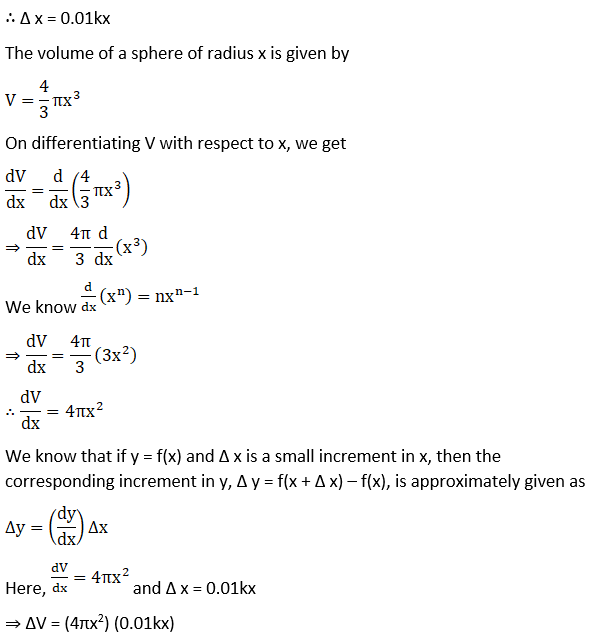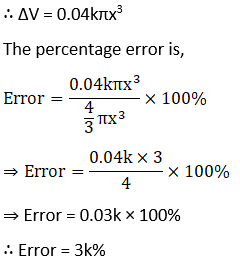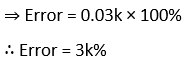## Frequently Asked Questions on RD Sharma Solutions for Class 12 Maths Chapter 14

### What are the benefits of referring to the RD Sharma Solutions for Class 12 Maths Chapter 14?

Every student has to take up exams as they are the basis of assessing how knowledgeable and informational a student is. The RD Sharma Solutions for Class 12 Maths Chapter 14 provide precise and detailed answers to the textbook questions. Students can also improve their problem solving abilities and time management skills by regularly using these resources while preparing for exams. All the concepts are explained in simple steps to aid students to ace their annual exam.

### Why should students opt BYJU’S RD Sharma Solutions for Class 12 Maths Chapter 14 for reference?

Students should opt the BYJU’S RD Sharma Solutions for Class 12 Maths Chapter 14 as it’s handy and easy to understand. Moreover, the solutions can be viewed online and downloaded in PDF for offline use by the students for free and without any time constraints. All the solutions are prepared by subject experts at BYJU’S using simple language for the ease of learning. Neat explanations using steps in the solutions help students learn answer presentation skills vital for exams.

### Is sufficient information covered in the RD Sharma Solutions for Class 12 Maths Chapter 14?

Yes, the RD Sharma Solutions for Class 12 Maths Chapter 14 are well researched and presented by the academic experts, which makes it more informative and accurate from the exam point of view. By regular practice, students will be able to analyse how to present quality answers as per the marks weightage of the CBSE board. This increases the chances of performing well in the board exam with a good score.